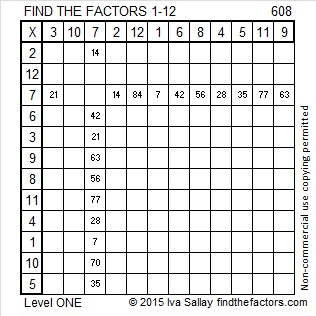# 608 and Level 1

608 is the sum of 19 consecutive numbers:

23 + 24 + 25 + 26 + 27 + 28 + 29 + 30 + 31 + 32 +33 + 34 + 35 + 36 + 37 + 38 + 39 + 40 + 41 = 608. What does that have to do with the factors of 608?Print the puzzles or type the solution on this excel file: 12 Factors 2015-09-07

—————————————————————————————————

• 608 is a composite number.
• Prime factorization: 608 = 2 x 2 x 2 x 2 x 2 x 19, which can be written 608 = (2^5) x 19
• The exponents in the prime factorization are 5 and 1. Adding one to each and multiplying we get (5 + 1)(1 + 1) = 6 x 2 = 12. Therefore 608 has exactly 12 factors.
• Factors of 608: 1, 2, 4, 8, 16, 19, 32, 38, 76, 152, 304, 608
• Factor pairs: 608 = 1 x 608, 2 x 304, 4 x 152, 8 x 76, 16 x 38, or 19 x 32
• Taking the factor pair with the largest square number factor, we get √608 = (√16)(√38) = 4√38 ≈ 24.657656.—————————————————————————————————This site uses Akismet to reduce spam. Learn how your comment data is processed.